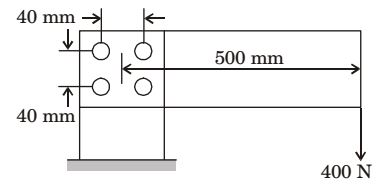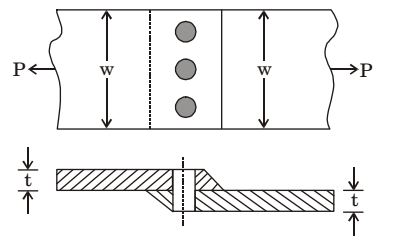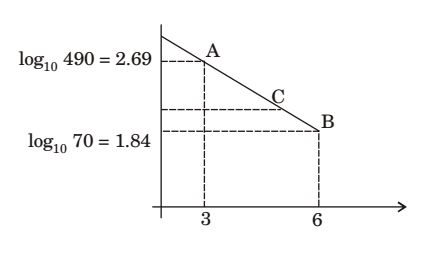## Machine Design Miscellaneous

#### Machine Design

1. In order to test the efficiency of reducer gear train 1 kW input was given at the input end at a speed of 1440 rpm and at the output end the measured torque was 56.36 Nm. If the ratio of speed reduction in this unit is 10: 1, the efficiency is about

1. Speed reduction = 10: 1

 Output speed = 1440 = 144 rpm 10

 Output Torque = T.ω = 56.36 × 2π × 144 = 849.88 N - m 60

 % efficiency = Output × 100 Input

 = 849.88 × 100 = 85 %(Approximately) 1000

##### Correct Option: B

Speed reduction = 10: 1

 Output speed = 1440 = 144 rpm 10

 Output Torque = T.ω = 56.36 × 2π × 144 = 849.88 N - m 60

 % efficiency = Output × 100 Input

 = 849.88 × 100 = 85 %(Approximately) 1000

1. Which of the bearings given below SHOULD NOT be subjected to a thrust load?

1. NA

##### Correct Option: C

NA

1. A horizontal plate has been joined to a vertical to a vertical post using four rivets arranged as shown in the figure. The magnitude of the load on the worst loaded rivet (in N) is _______.1. Primary force (P1) = 100 N

 P"1 = P"2 = P"3 = P"4 = Pe × r 4r²

 = 400 × 500 ×40√22 4 ×40√22

= P"1 = 1767.766N
1 & 4 are worst loaded
∴ (Pnet)² = (P1)² + (P"2)² + 2(P1)(P"1) cos 45°
Pnet = 1839.83 N

##### Correct Option: C

Primary force (P1) = 100 N

 P"1 = P"2 = P"3 = P"4 = Pe × r 4r²

 = 400 × 500 ×40√22 4 ×40√22

= P"1 = 1767.766N
1 & 4 are worst loaded
∴ (Pnet)² = (P1)² + (P"2)² + 2(P1)(P"1) cos 45°
Pnet = 1839.83 N

Direction: A single riveted lap Joint of two similar plates as shown in the figure below has the following geometrical and material details.width of the plate ω = 200 mm, thickness of the plate t = 5 mm, number of rivets n = 3, diameter of the rivet dr = 10 mm, diameter of the rivet hole dr= 11 M mm, allowable tensile stress of the plate σp = 200 MPa, allowable shear stress of the rivet σs = 100 MPa and allowable bearing stress of the rivet σc = 150 MPa.

1. If the rivets are to be designed to avoid crushing failure, the maximum permissible load P in kN is

1. For rivet,

 σ P = P 3Ar 3d × t

∴ Ps = 100 × d × t × 3 = 15 kN
and Pc = 150 × d × t × 3 = 22.5 kN
∴ p = max (15, 22.5) = 22.5 kN

##### Correct Option: C

For rivet,

 σ P = P 3Ar 3d × t

∴ Ps = 100 × d × t × 3 = 15 kN
and Pc = 150 × d × t × 3 = 22.5 kN
∴ p = max (15, 22.5) = 22.5 kN

1. A cylindrical shaft is subjected to an alternating stress of 100 MPa. Fatigue strength to sustain 1000 cycles is 490 MPa. If the corrected endurance strength is 70 MPa, estimated shaft life will be

1.By concept of straight line,

 y - 1.8451 = 1.8451 - 2.6902 x - 6 6 - 3

At y = 2
⇒ x = 5.4501
log10N = 5.4501, N = 281914

##### Correct Option: CBy concept of straight line,

 y - 1.8451 = 1.8451 - 2.6902 x - 6 6 - 3

At y = 2
⇒ x = 5.4501
log10N = 5.4501, N = 281914